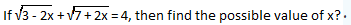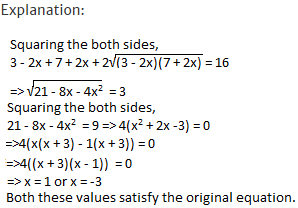Courses

## 10 Questions MCQ Test Quantitative Aptitude (Quant) | Test: Quadratic Equations- 2

Description
This mock test of Test: Quadratic Equations- 2 for Banking Exams helps you for every Banking Exams entrance exam. This contains 10 Multiple Choice Questions for Banking Exams Test: Quadratic Equations- 2 (mcq) to study with solutions a complete question bank. The solved questions answers in this Test: Quadratic Equations- 2 quiz give you a good mix of easy questions and tough questions. Banking Exams students definitely take this Test: Quadratic Equations- 2 exercise for a better result in the exam. You can find other Test: Quadratic Equations- 2 extra questions, long questions & short questions for Banking Exams on EduRev as well by searching above.
QUESTION: 1

### Find the value of a/b + b/a, if a and b are the roots of the quadratic equation x2 + 8x + 4 = 0?

Solution:

Explanation:

a/b + b/a = (a2 + b2)/ab = (a2 + b2 + a + b)/ab
= [(a + b)2 - 2ab]/ab
a + b = -8/1 = -8
ab = 4/1 = 4
Hence a/b + b/a = [(-8)2 - 2(4)]/4 = 56/4 = 14.

QUESTION: 2

###Solution:QUESTION: 3

### Find the quadratic equations whose roots are the reciprocals of the roots of 2x2 + 5x + 3 = 0?

Solution:

Explanation:

The quadratic equation whose roots are reciprocal of 2x2 + 5x + 3 = 0 can be obtained by replacing x by 1/x.
Hence, 2(1/x)2 + 5(1/x) + 3 = 0
=> 3x2 + 5x + 2 = 0

QUESTION: 4

A man could buy a certain number of notebooks for Rs.300. If each notebook cost is Rs.5 more, he could have bought 10 notebooks less for the same amount. Find the price of each notebook?

Solution:

Explanation:

Let the price of each note book be Rs.x.
Let the number of note books which can be brought for Rs.300 each at a price of Rs.x be y.
Hence xy = 300
=> y = 300/x
(x + 5)(y - 10) = 300 => xy + 5y - 10x - 50 = xy
=>5(300/x) - 10x - 50 = 0 => -150 + x2 + 5x = 0
multiplying both sides by -1/10x
=> x2 + 15x - 10x - 150 = 0
=> x(x + 15) - 10(x + 15) = 0
=> x = 10 or -15
As x>0, x = 10.

QUESTION: 5

I. a2 - 7a + 12 = 0,
II. b2 - 3b + 2 = 0 to solve both the equations to find the values of a and b?

Solution:

Explanation:

I.(a - 3)(a - 4) = 0
=> a = 3, 4

II. (b - 2)(b - 1) = 0
=> b = 1, 2
=> a > b

QUESTION: 6

I. a2 - 9a + 20 = 0,
II. 2b2 - 5b - 12 = 0 to solve both the equations to find the values of a and b?

Solution:

Explanation:

I. (a - 5)(a - 4) = 0
=> a = 5, 4
II. (2b + 3)(b - 4) = 0
=> b = 4, -3/2 => a ≥ b

QUESTION: 7

I. a2 + 11a + 30 = 0,
II. b2 + 6b + 5 = 0 to solve both the equations to find the values of a and b?

Solution:

Explanation:

I. (a + 6)(a + 5) = 0
=> a = -6, -5
II. (b + 5)(b + 1) = 0
=> b = -5, -1 => a ≤ b

QUESTION: 8

I. a2 + 8a + 16 = 0,
II. b2 - 4b + 3 = 0 to solve both the equations to find the values of a and b?

Solution:

Explanation:

I. (a + 4)2 = 0 => a = -4
II.(b - 3)(b - 1) = 0
=> b = 1, 3 => a < b

QUESTION: 9

I. a2 - 2a - 8 = 0,
II. b2 = 9 to solve both the equations to find the values of a and b?

Solution:

Explanation:

I. (a - 4)(a + 2) = 0
=> a = 4, -2
II. b2 = 9
=> b = ± 3
-2 < 3, -2 > -3, 4 > 3, 4 > -3,
No relation can be established between a and b.

QUESTION: 10

I. x2 + 5x + 6 = 0,
II. y2 + 9y +14 = 0 to solve both the equations to find the values of x and y?

Solution:

I. x2 + 3x + 2x + 6 = 0
=> (x + 3)(x + 2) = 0 => x = -3 or -2
II. y2 + 7y + 2y + 14 = 0
=> (y + 7)(y + 2) = 0 => y = -7 or -2
No relationship can be established between x and y.